# Basic Principles¶

Purpose: This section provides the key components to understand the geophysical magnetic experiment. As briefly summarized in the Introduction section, the magnetic survey requires a magnetic source. Rocks inside the earth become magnetized and they produce an anomalous magnetic field data. A receiver records the sum of all magnetic fields.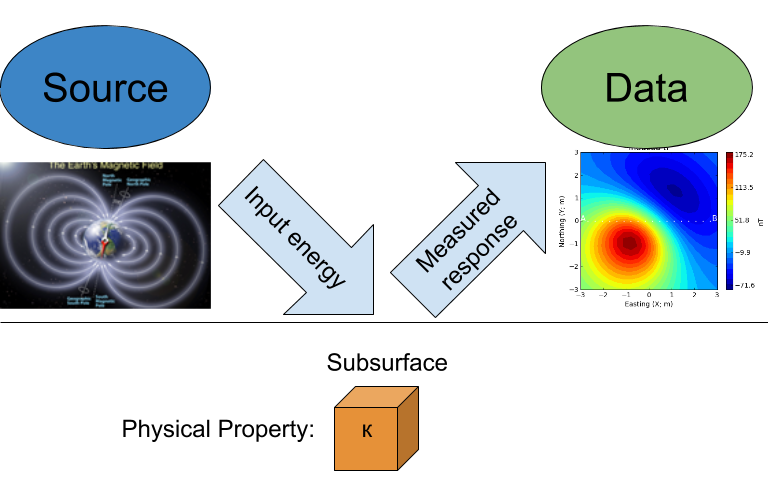Important topics are:

## Earth’s magnetic field (Source)¶All magnetic fields arise from currents. This is also true for the magnetic field of the earth. The outer core of the earth is molten and in a state of convection and a geomagnetic dynamo creates magnetic fields. Close to the surface of the core the magnetic fields are very complicated but as we move outward the magnetic field resembles that of a large bar magnetic which is often referred to as a magnetic dipole.To a first approximation, Earth’s magnetic field resembles a large dipolar source with a negative pole in the northern hemisphere and a positive pole in the southern hemisphere (Fig. 24). The dipole is offset from the center of the earth and also tilted. The north magnetic pole at the surface of the earth is approximately at Melville Island.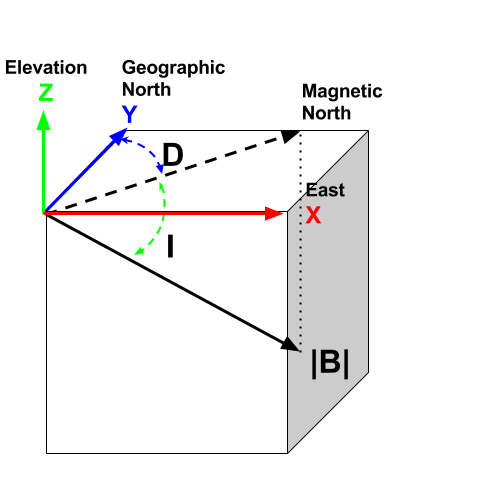The field at any location on (or above or within) the Earth are generally described in terms described of magnitude ($$\mathbf{|B|}$$), declination ($$\mathbf{D}$$) and inclination ($$\mathbf{I}$$) as illustrated in Fig. 25.

• $$\mathbf{|B|}$$: The magnitude of the vector representing Earth’s magnetic field.
• $$\mathbf{D}$$: Declination is the angle that H makes with respect to geographic north (positive angle clockwise).
• $$\mathbf{I}$$: Inclination is the angle between B and the horizontal. It can vary between -90° and +90° (positive angle down).

### The IGRF¶

Earth’s field at any location is approximately that provided by a global reference model called the IGRF or International Geomagnetic Reference Field. The IGRF is a mathematical model that describes the field and its secular changes, that is, how it changes with time. The IGRF is a product of the International Association of Geomagnetism and Aeronomy (IAGA), and the original version was defined in 1968. It is updated every five years, and later versions may re-define the field at earlier times. This is important to remember if you are comparing old maps to new ones.

Earth’s field has a strength of approximately 70,000 nanoTeslas (nT) at the magnetic poles and approximately 25,000 nT at the magnetic equator. Field orientation and strength varies around the world, as presented in Table 2 based upon the IGRF from 2003 (NOAA).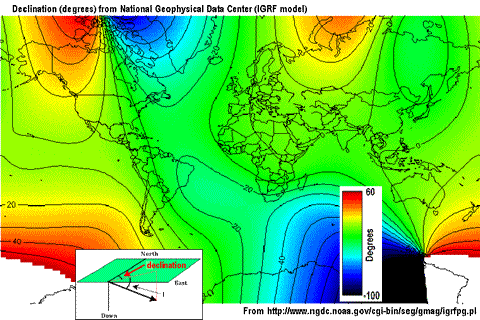Fig. 26 Earth’s declination.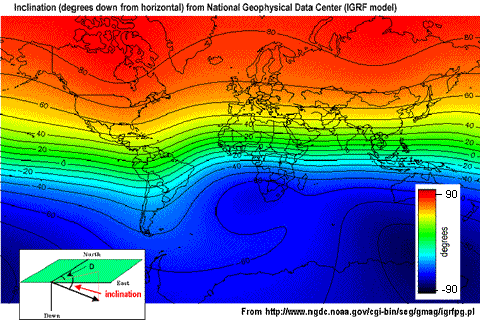Fig. 27 Earth’s inclination.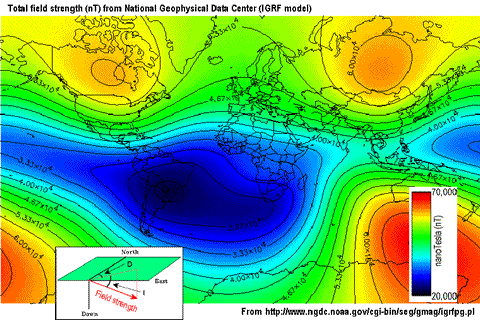Fig. 28 Earth’s field strentgh

Slow changes in the exact location of the magnetic north pole occur over long periods (months-years). These changes are thought to be caused by internal changes in mantle convection. Knowing the acquisition date of a magnetic survey is important in order to understand the observed magnetic anomalies. In 2004, Earth’s magnetic north pole was close to Melville Island (Nunavut) at (Latitude, Longitude)=(79N, 70W). In Vancouver (BC), the current field is orientated at D ~ 20°N, ~ 70° Inclination. Various governmental agencies are actively collecting and archiving information about the parameters of the field worldwide and can be queried with the magnetic field calculator.

Details about Earth’s field can be found at government geoscience websites such as the NOAA geomagnetism home page, or the Canadian National Geomagnetism Program home page. An overview of Earth’s magnetic field (with good images, graphs, etc.) can be found on the British Geological Survey’s geomagnetics website.

### Magnetic fields from External Sources¶

When we record a magnetic observation we measure the field that exists at that location. Most of that field comes from inside the earth and it can be from the geomagnetic dynamo or from crustal rocks that have become magnetized. In addition there are also magnetic fields that come from outside the earth. The solar wind interacts with Earth’s magnetic field and creates a magnetosphere that is “tear-dropped” shape as shown in the figure below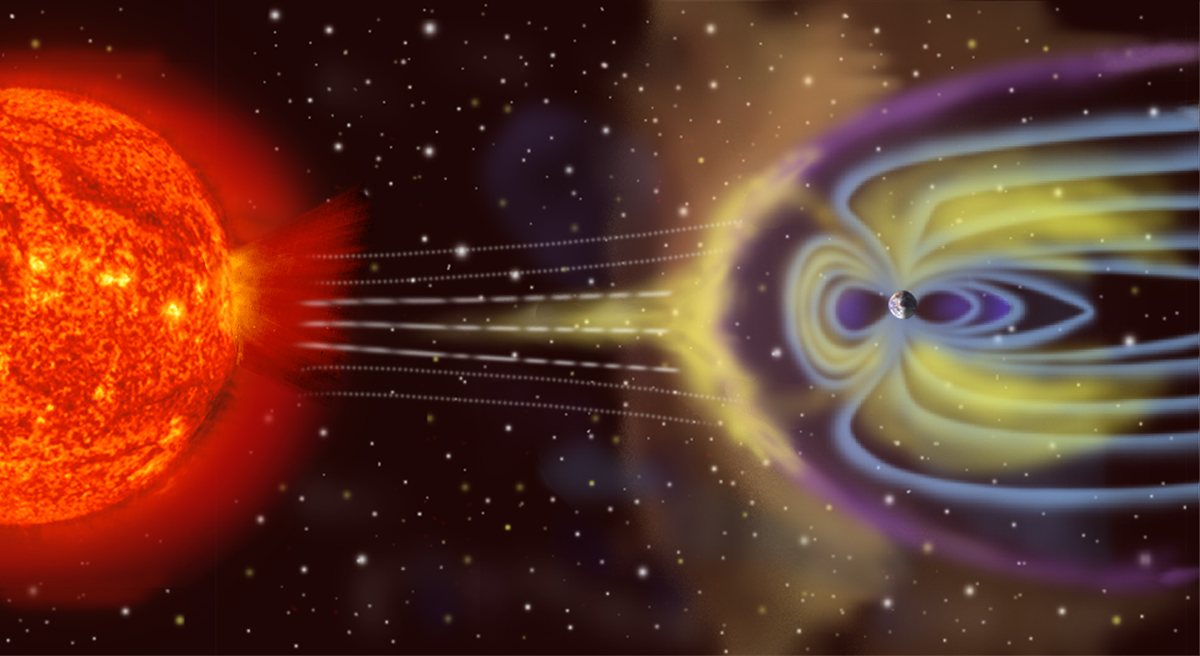Fig. 29 The image shows an artist’ rendition of the charged particles interacting with Earth’s magnetic field. The volume containing Earth’s field is called the magnetosphere (by_NASA).

The interaction between Earth’s field and the solar wind allows charge particles to flow in the ionsphere which is a zone of ionized particles about 110 km above the earth’s surface. These currents produce magnetic fields. The time-scales for these changes can be very short, in the order of micro- seconds, to large, in the order of days. Daily variations can typically be on the order of 20 - 50 nT in size. Large scale variations are caused by magnetic storms and they may be 1000’s of nT in size. Magnetic storms are correlated with sunspot activity, usually on an 11-year cycle. These variations can be large enough to cause damage to satellites and power distribution systems. They are also the cause of the Aurora Borealis or Australis (northern or southern lights respectively). See the GSC’s “Geomagnetic Hazards” web page for more.

## Magnetization¶

When the source field is applied to earth materials it causes the to become magnetized. Magnetization is the dipole moment per unit volume. This is a vector quantity because a dipole has a strength and a direction. For many cases of interest the relationship between magnetization $$\mathbf{M}$$ and the source $$\mathbf{H}$$ (earth’s magnetic field) is given by

(1)$\mathbf{M} = \kappa \mathbf{H}.$

where $$\kappa$$ is the magnetic susceptibility. Thus the magnetization has the same direction as the earth’s field. Because Earth’s field is different at different locations on the earth, then the same object gets magnetized differently depending upon where it is situated. As a consequence, magnetic data from a steel drum buried at the north pole will be very different from that from a drum buried at the equator.

The final magnetization of a rock or man-made object can be the result of a number of contributing factors. In the case of the metal drum, it can made of steel and it has complicated structure. It’s walls are thin, it is hollow on the inside, and the steel has a very high magnetic susceptibility. The geometry and high susceptibility causes the induced magnetic field of the drum to be in a different direction than the inducing earth’s field and the relationship (1) is no longer valid. Also, the drum was manufactured by molding melted steel. When that material cooled through its Curie temperature it acquired a permanent, or remanent, magnetization. It’s net magnetization, when it is buried at any location on the earth will be the sum of the induced and remanent magnetizations. This is an important topic and it is further investigated here.

## Responses (Data)¶

The magnetic field that results from the magnetized earth is evaluated with the equation

(2)$\mathbf{B}_A = \frac{\mu_0}{4\pi} \int_{V} \mathbf{M} \cdot \nabla \nabla \left(\frac{1}{r}\right) \; dV \;,$

where $$\mu_0$$ is the magnetic permeability of free space, $$\mathbf{M}$$ is the magnetization per unit volume $$\mathbf{V}$$, and $$r$$ defines the distance between the object and the location of the observer. This magnetic field is referred to as the “secondary” field or sometimes the “anomalous” field $$\mathbf{B}_A$$. For geological or engineering problems, these anomalous fields are the data to be interpreted, and this is what we seek to measure.

When the magnetization is governed by the linear relationship (1) then the above anomalous field can be written as:

(3)$\mathbf{B}_A = \frac{\mu_0}{4\pi} \int_{V} \kappa \mathbf{H}_0 \cdot \nabla \nabla \left(\frac{1}{r}\right) \; dV \;.$

It is important to note that the left hand side of this equation is a magnetic field that is a vector. For simplicity, and for the remainder of this section, we shall drop the subscript “A” and remember that we are talking about anomalous fields. A vector in three dimensional space requires three numbers to specify it. These could be component values ($$B_x,\;B_y,\;B_z$$) or an amplitude and angles ( $$|B|,\;D,\;I$$). Generally a geophysical datum is a measurement of a component. For instance,

$B_x = \mathbf{B} \cdot \mathbf{\hat x} \;,$

where ($$\cdot$$) is a vector inner product. This means that $$B_x$$ is the projection of the vector $$\mathbf{B}$$ onto a unit vector in the $$\mathbf{\hat x}$$ direction. Similar understandings exist for $$B_y$$ and $$B_z$$. When plotting magnetic field data over an object it is therefore usual to plot maps of a particular component. A special datum that is particularly important for magnetics is the projection of the anomalous field onto a unit vector that is in the direction of the earth’s field. Let this be $$\mathbf{\hat B_0}$$. Then the datum $$\mathbf{B_t}$$ is

$\mathbf{B_t} = \mathbf{B} \cdot \mathbf{\hat B_0}$

The basic ideas behind the induced magnetization process, going from source to data, are illustrated below. The image of the data, corresponds to $$\mathbf{B_t}$$.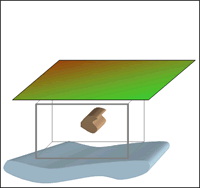1. No incident magnetic field.

2. Earth's field causes material to become magnetized.

3. Plotting these measurements produces a map of this magnetic anomaly.

4. Often measurements are made along lines and the anomaly is plotted as a graph.

From (3), we note that the induced response of the field will vary both in magnitude and orientation with respect to the inducing magnetic field $$\mathbf{H}_0$$. Therefore, the magnetic response of an object buried in Canada may look a lot different if buried near the equator as demonstrated in the dipole animation below. This is an important point to keep in mind when interpreting magnetic data.

 Once Loop Reflect